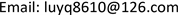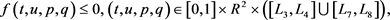﻿ 障碍带条件下两类三阶非线性边值问题解的存在性 Existence of Solutions for Two Class of Third-Order Nonlinear Boundary Value Problems under Barrier Strips Conditions

Pure Mathematics
Vol.08 No.03(2018), Article ID:25041,8 pages
10.12677/PM.2018.83030

Existence of Solutions for Two Class of Third-Order Nonlinear Boundary Value Problems under Barrier Strips Conditions

Dongmei Zhang, Yanqiong Lu

College of Mathematics and Statistics, Northwest Normal University, Lanzhou GansuReceived: May 2nd, 2018; accepted: May 15th, 2018; published: May 24th, 2018ABSTRACT

By using Leary-Schauder theorem, we study the existence of solutions for two classes of nonlinear third-order two-point boundary value problems. We establish the existence results of solutions for the above two classes of boundary value problems with nonlinearity satisfying barrier strips conditions and give some examples to illustrate our main results.

Keywords:Barrier Strips Conditions, Third-Order Boundary Value Problems, Existence, Leary-Schauder TheoremCopyright © 2018 by authors and Hans Publishers Inc.

This work is licensed under the Creative Commons Attribution International License (CC BY).

http://creativecommons.org/licenses/by/4.0/1. 引言

1994年，P. Kelevedjiev  运用Leray-Schauder原理证明了障碍带条件下非线性二阶两点边值问题

$\left\{\begin{array}{l}-{u}^{″}\left(t\right)=f\left(t,u,{u}^{\prime }\right),\text{\hspace{0.17em}}\text{\hspace{0.17em}}t\in \left[0,1\right],\\ u\left(0\right)=A,\text{\hspace{0.17em}}\text{\hspace{0.17em}}\text{\hspace{0.17em}}\text{\hspace{0.17em}}u\left(0\right)=B\end{array}$

$\left\{\begin{array}{l}-{u}^{‴}\left(t\right)=f\left(t,u,{u}^{\prime },{u}^{″}\right),\text{\hspace{0.17em}}\text{\hspace{0.17em}}t\in \left[0,1\right],\\ u\left(0\right)={u}^{\prime }\left(0\right)={u}^{″}\left(1\right)=0\end{array}$ (1)

2009年，张宏旺  运用新的极大值原理及上下解的单调迭代法获得了非线性三阶边值问题

$\left\{\begin{array}{l}-{u}^{‴}\left(t\right)=f\left(t,u\left(t\right)\right),\text{\hspace{0.17em}}\text{\hspace{0.17em}}t\in \left[0,1\right],\\ u\left(0\right)={u}^{\prime }\left(0\right)=u\left(1\right)=0\end{array}$

$\left\{\begin{array}{l}-{u}^{‴}\left(t\right)=f\left(t,u\left(t\right),{u}^{\prime }\left(t\right),{u}^{″}\left(t\right)\right),\text{\hspace{0.17em}}\text{\hspace{0.17em}}t\in \left(0,1\right),\\ u\left(0\right)={u}^{\prime }\left(0\right)=u\left(1\right)=0\end{array}$

$\left\{\begin{array}{l}{u}^{‴}\left(t\right)=f\left(t,u\left(t\right),{u}^{\prime }\left(t\right),{u}^{″}\left(t\right)\right),\text{\hspace{0.17em}}\text{\hspace{0.17em}}t\in \left[0,1\right],\\ u\left(0\right)=A,\text{\hspace{0.17em}}u\left(1\right)=B,\text{\hspace{0.17em}}{u}^{\prime }\left(1\right)=C\end{array}$ (2)

$\left\{\begin{array}{l}{u}^{‴}\left(t\right)=f\left(t,u\left(t\right),{u}^{\prime }\left(t\right),{u}^{″}\left(t\right)\right),\text{\hspace{0.17em}}\text{\hspace{0.17em}}t\in \left[0,1\right],\\ u\left(0\right)=A,\text{\hspace{0.17em}}u\left(1\right)=B,\text{\hspace{0.17em}}{u}^{\prime }\left(0\right)=C\end{array}$ (3)

2. 预备知识

$C\left[0,1\right]$ 表示区间 $\left[0,1\right]$ 上连续函数构成的空间，定义其上范数为 ${‖u‖}_{0}=\mathrm{max}\left\{|u\left(t\right)|:t\in \left[0,1\right]\right\}$ 。记 ${C}^{3}\left[0,1\right]=\left\{u\text{\hspace{0.17em}}|\text{\hspace{0.17em}}u,{u}^{\prime },{u}^{″},{u}^{‴}\in C\left[0,1\right]\right\}$ ，其上范数为：

${‖u‖}_{3}=\mathrm{max}\left\{{‖u‖}_{0},{‖{u}^{\prime }‖}_{0},{‖{u}^{″}‖}_{0},{‖{u}^{‴}‖}_{0}\right\},$

${C}^{3}\left[0,1\right]$ 按范数 ${‖u‖}_{3}$ 构成Banach空间。

${A}_{1}=\left\{u\in C\left[0,1\right]|u\left(0\right)=A,u\left(1\right)=B,{u}^{\prime }\left(1\right)=C,A,B,C为常数\right\},$

${A}_{2}=\left\{u\in C\left[0,1\right]|u\left(0\right)=A,u\left(1\right)=B,{u}^{\prime }\left(0\right)=C,A,B,C为常数\right\}$

${T}_{i}u={u}^{‴},\text{\hspace{0.17em}}i=1,2.$

$\left\{u|u\in E,u=\lambda Au,0<\lambda <1\right\}$

3. 主要结果及证明

${L}_{2}>{L}_{1}\ge D,{L}_{4}>{L}_{3}\ge D,{L}_{5}<{L}_{6}\le D,{L}_{8}<{L}_{7}\le D,$

$f\left(t,u,p,q\right)\ge 0,\text{\hspace{0.17em}}\left(t,u,p,q\right)\in \left[0,1\right]×{R}^{2}×\left(\left[{L}_{1},{L}_{2}\right]\cup \left[{L}_{5},{L}_{6}\right]\right),$$\left\{\begin{array}{l}{u}^{‴}\left(t\right)=\lambda f\left(t,u\left(t\right),{u}^{\prime }\left(t\right),{u}^{″}\left(t\right)\right),t\in \left[0,1\right],\lambda \in \left[0,1\right],\\ u\left(0\right)=A,u\left(1\right)=B,{u}^{\prime }\left(1\right)=C.\end{array}$ (4)

$u\in {C}^{3}\left[0,1\right]$ 是问题(4)的一个解。则由微分中值定理得，存在 $d\in \left(0,1\right)$ ，使得 ${u}^{\prime }\left(d\right)=u\left(1\right)-u\left(0\right)=B-A$ 。同理，存在 $\xi \in \left(d,1\right)$ ，使得

${u}^{″}\left(\xi \right)=\frac{{u}^{\prime }\left(1\right)-{u}^{\prime }\left(d\right)}{1-d}=\frac{C-B+A}{1-d}=D.$

$f\left(t,u,p,q\right)\ge 0,\left(t,u,p,q\right)\in \left[0,\xi \right]×{R}^{2}×\left[{L}_{1},{L}_{2}\right],$

$f\left(t,u,p,q\right)\le 0,\left(t,u,p,q\right)\in \left[0,\xi \right]×{R}^{2}×\left[{L}_{7},{L}_{8}\right].$

${S}_{0}=\left\{t\in \left[0,\xi \right]:{L}_{1}<{u}^{″}\left(t\right)\le {L}_{2}\right\},\text{\hspace{0.17em}}\text{\hspace{0.17em}}{S}_{1}=\left\{t\in \left[0,\xi \right]:{L}_{7}<{u}^{″}\left(t\right)\le {L}_{8}\right\}$

${u}^{″}\left({{t}^{\prime }}_{0}\right)<{u}^{″}\left({t}_{0}\right),\text{\hspace{0.17em}}\text{\hspace{0.17em}}{u}^{″}\left({{t}^{\prime }}_{1}\right)>{u}^{″}\left({t}_{1}\right).$ (5)

${u}^{″}$ 的连续性，甚至可以取到 ${{t}^{\prime }}_{0}\in \left({t}_{0},\xi \right]\cap {S}_{0}$${{t}^{\prime }}_{1}\in \left({t}_{1},\xi \right]\cap {S}_{1}$ ，但对 $t\in {S}_{0}$ ，有

${u}^{‴}\left(t\right)=\lambda f\left(t,u\left(t\right),{u}^{\prime }\left(t\right),{u}^{″}\left(t\right)\right)\ge 0,$

${u}^{″}\left({{t}^{\prime }}_{0}\right)\ge {u}^{″}\left({t}_{0}\right),\text{\hspace{0.17em}}t\in \left({t}_{0},\xi \right],\text{\hspace{0.17em}}\text{\hspace{0.17em}}{u}^{″}\left({{t}^{\prime }}_{1}\right)\le {u}^{″}\left({t}_{1}\right),\text{\hspace{0.17em}}t\in \left({t}_{1},\xi \right].$

$|{u}^{″}\left(t\right)|\le \mathrm{max}\left\{|{L}_{1}|,|{L}_{8}|\right\},t\in \left[0,\xi \right].$

$f\left(t,u,p,q\right)\le 0,\text{\hspace{0.17em}}\left(t,u,p,q\right)\in \left[\xi ,1\right]×{R}^{2}×\left[{L}_{3},{L}_{4}\right],$

$f\left(t,u,p,q\right)\ge 0,\text{\hspace{0.17em}}\left(t,u,p,q\right)\in \left[\xi ,1\right]×{R}^{2}×\left[{L}_{5},{L}_{6}\right]$

$|{u}^{″}\left(t\right)|\le {M}_{0},$ (6)

${u}^{\prime }\left(1\right)-{u}^{\prime }\left(t\right)={u}^{″}\left(\tau \right)\left(1-t\right)$

$|{u}^{\prime }\left(t\right)|\le {M}_{1},\text{\hspace{0.17em}}t\in \left[0,1\right],$ (7)

$u\left(t\right)-u\left(0\right)={u}^{\prime }\left(\rho \right)t,$

$|u\left(t\right)|\le {M}_{2},\text{\hspace{0.17em}}t\in \left[0,1\right],$ (8)

$|{u}^{‴}\left(t\right)|\le {M}_{3},\text{\hspace{0.17em}}t\in \left[0,1\right],$ (9)

${‖u‖}_{3}<\mathrm{max}\left\{{M}_{0},{M}_{1},{M}_{2},{M}_{3}\right\}+1.$

${L}_{2}>{L}_{1}\ge D,{L}_{4}>{L}_{3}\ge D,{L}_{5}<{L}_{6}\le D,{L}_{8}<{L}_{7}\le D$ ，其中 $D=\frac{C-B+A}{1-d},d\in \left(0,1\right)$ ，满足

$f\left(t,u,p,q\right)\le 0,\text{\hspace{0.17em}}\left(t,u,p,q\right)\in \left[0,1\right]×{R}^{2}×\left(\left[{L}_{1},{L}_{2}\right]\cup \left[{L}_{5},{L}_{6}\right]\right),$

$f\left(t,u,p,q\right)\ge 0,\text{\hspace{0.17em}}\left(t,u,p,q\right)\in \left[0,1\right]×{R}^{2}×\left(\left[{L}_{3},{L}_{4}\right]\cup \left[{L}_{7},{L}_{8}\right]\right),$

$\underset{_}{L}<0<\overline{L}$ ，使得

$f\left(t,u,p,q\right)\ge 0,\text{\hspace{0.17em}}\left(t,u,p,q\right)\in \left[0,1\right]×{R}^{2}×\left\{\overline{L}\right\},$

$f\left(t,u,p,q\right)\le 0,\text{\hspace{0.17em}}\left(t,u,p,q\right)\in \left[0,1\right]×{R}^{2}×\left\{\underset{_}{L}\right\},$

$\varphi \left(\overline{L}\right)=1,\text{\hspace{0.17em}}\varphi \left(\underset{_}{L}\right)=-1.$

$\left\{\begin{array}{l}{u}^{‴}\left(t\right)={f}_{m}\left(t,u,{u}^{\prime },{u}^{″}\right),t\in \left[0,1\right],\\ u\left(0\right)=A,u\left(1\right)=B,{u}^{\prime }\left(1\right)=C\end{array}$ (10)

${f}_{m}\left(t,u,p,q\right)>0,\text{\hspace{0.17em}}\left(t,u,p,q\right)\in \left[0,1\right]×{R}^{2}×\left[{L}_{m,1},{L}_{m,2}\right].$

${f}_{m}\left(t,u,p,q\right)<0,\text{\hspace{0.17em}}\left(t,u,p,q\right)\in \left[0,1\right]×{R}^{2}×\left[{L}_{m,3},{L}_{m,4}\right].$

$\left[{L}_{m+1,1},{L}_{m+1,2}\right]\subseteq \left[{L}_{m,1},{L}_{m,2}\right],$ (11)

$\left[{L}_{m+1,3},{L}_{m+1,4}\right]\subseteq \left[{L}_{m,3},{L}_{m,4}\right],$ (12)

${L}_{m,4}\le {u}^{″}\left(t\right)\le {L}_{m,1},\text{\hspace{0.17em}}t\in \left[0,1\right].$

$|{{u}^{″}}_{m}\left(t\right)|\le {M}_{4},\text{\hspace{0.17em}}t\in \left[0,1\right].$ (13)

${{u}^{\prime }}_{m}\left(t\right)-{{u}^{\prime }}_{m}\left(0\right)={{u}^{″}}_{m}\left(\eta \right)t,$

$|{{u}^{\prime }}_{m}\left(t\right)|\le {M}_{5},\text{\hspace{0.17em}}t\in \left[0,1\right].$ (14)

$|{u}_{m}\left(t\right)|\le {M}_{6},\text{\hspace{0.17em}}t\in \left[0,1\right].$ (15)

$|{{u}^{‴}}_{m}\left(t\right)|\le {M}_{7},\text{\hspace{0.17em}}t\in \left[0,1\right].$ (16)

$\underset{i\to \infty }{\mathrm{lim}}{u}_{{m}_{i}}=\nu \in C\left[0,1\right].$

$\left\{{u}_{{m}_{i}}\right\}$ 有界，且 ${C}^{3}\left[0,1\right]$ 紧嵌入 ${C}^{1}\left[0,1\right]$ ，故存在子序列 $\left\{{u}_{{m}_{{i}_{j}}}\right\}\subseteq \left\{{u}_{m}\right\}$ ，满足

$\underset{i\to \infty }{\mathrm{lim}}{u}_{{m}_{{}_{{i}_{j}}}}=\nu ,\text{\hspace{0.17em}}\underset{j\to \infty }{\mathrm{lim}}{{u}^{\prime }}_{{m}_{{i}_{j}}}={\nu }^{\prime },$

$\underset{i\to \infty }{\mathrm{lim}}{u}_{{m}_{{}_{{i}_{{j}_{k}}}}}=\nu ,\text{\hspace{0.17em}}\underset{j\to \infty }{\mathrm{lim}}{{u}^{\prime }}_{{m}_{{i}_{j}{}_{k}}}={\nu }^{\prime },\text{\hspace{0.17em}}\underset{k\to \infty }{\mathrm{lim}}{{u}^{″}}_{{m}_{{i}_{{j}_{k}}}}={\nu }^{″}.$

$\left\{\begin{array}{l}{{\theta }^{‴}}_{m}\left(t\right)={f}_{m}\left(t,{\theta }_{m},{{\theta }^{\prime }}_{m},{{\theta }^{″}}_{m}\right),t\in \left[0,1\right],\\ {\theta }_{m}\left(0\right)=A,{\theta }_{m}\left(1\right)=B,{{\theta }^{\prime }}_{m}\left(0\right)=C,\end{array}$ (17)

${\theta }_{m}\left(t\right)={\int }_{0}^{1}G\left(t,s\right){f}_{m}\left(s,{\theta }_{m}\left(s\right),{{\theta }^{\prime }}_{m}\left(s\right),{{\theta }^{″}}_{m}\left(s\right)\right)\text{d}s+\left(B-A-C\right){t}^{2}+Ct+A,$ (18)

$G\left(t,s\right)=\left\{\begin{array}{l}\frac{{t}^{2}{\left(1-s\right)}^{2}}{2},\text{\hspace{0.17em}}0\le t\le s\le 1\\ \frac{\left(1-t\right)s\left(t-s+t\left(1-s\right)\right)}{2},\text{\hspace{0.17em}}0\le s\le t\le 1,\end{array}$

$f\left(t,u,p,q\right)\le 0,\text{\hspace{0.17em}}\left(t,u,p,q\right)\in \left[0,1\right]×{R}^{2}×\left\{\overline{L}\right\},$

$f\left(t,u,p,q\right)\ge 0,\text{\hspace{0.17em}}\left(t,u,p,q\right)\in \left[0,1\right]×{R}^{2}×\left\{\underset{_}{L}\right\},$

${\theta }_{m}\left(t\right)={\int }_{0}^{1}G\left(t,s\right){f}_{m}\left(s,{\theta }_{m}\left(s\right),{{\theta }^{\prime }}_{m}\left(s\right),{{\theta }^{″}}_{m}\left(s\right)\right)\text{d}s+\left(C-B+A\right){t}^{2}+\left(2B-2A-C\right)t+A,$

$G\left(t,s\right)=\left\{\begin{array}{l}\frac{{\left(1-t\right)}^{2}{s}^{2}}{2},\text{\hspace{0.17em}}\text{\hspace{0.17em}}\text{\hspace{0.17em}}\text{\hspace{0.17em}}\text{\hspace{0.17em}}\text{\hspace{0.17em}}\text{\hspace{0.17em}}\text{\hspace{0.17em}}\text{\hspace{0.17em}}\text{\hspace{0.17em}}\text{\hspace{0.17em}}\text{\hspace{0.17em}}\text{\hspace{0.17em}}\text{\hspace{0.17em}}\text{\hspace{0.17em}}\text{\hspace{0.17em}}\text{\hspace{0.17em}}\text{\hspace{0.17em}}\text{\hspace{0.17em}}\text{\hspace{0.17em}}\text{\hspace{0.17em}}\text{\hspace{0.17em}}\text{\hspace{0.17em}}\text{\hspace{0.17em}}\text{\hspace{0.17em}}0\le s\le t\le 1;\\ \frac{\left(1-s\right)\left[\left(1-t\right)ts+t\left(s-t\right)\right]}{2},\text{\hspace{0.17em}}\text{\hspace{0.17em}}0\le t\le s\le 1\end{array}$

$\left\{\begin{array}{l}{u}^{‴}\left(t\right)=\frac{1}{5!}{\left({u}^{″}\left(t\right)-D\right)}^{5}-\frac{1}{3!}{\left({u}^{″}\left(t\right)-D\right)}^{3}+{u}^{″}\left(t\right)-D,\text{\hspace{0.17em}}t\in \left[0,1\right],\\ u\left(0\right)=A,\text{\hspace{0.17em}}\text{\hspace{0.17em}}u\left(1\right)=B,\text{\hspace{0.17em}}\text{\hspace{0.17em}}{u}^{\prime }\left(1\right)=C,\end{array}$ (19)$\left\{\begin{array}{l}{u}^{‴}\left(t\right)={\left({u}^{″}\left(t\right)-D\right)}^{3}+\frac{7}{10}{\left({u}^{″}\left(t\right)-D\right)}^{2}-\frac{9}{5}\left({u}^{″}\left(t\right)-D\right),\text{\hspace{0.17em}}t\in \left[0,1\right],\\ u\left(0\right)=A,\text{\hspace{0.17em}}\text{\hspace{0.17em}}u\left(1\right)=B,\text{\hspace{0.17em}}\text{\hspace{0.17em}}{u}^{\prime }\left(0\right)=C,\end{array}$ (20)

Existence of Solutions for Two Class of Third-Order Nonlinear Boundary Value Problems under Barrier Strips Conditions[J]. 理论数学, 2018, 08(03): 239-246. https://doi.org/10.12677/PM.2018.83030

1. 1. Grenier, W. (2004) Classical Mechanics-Point Particles and Relativity. Springer, Berlin.

2. 2. 杜睿娟. 障碍带条件下一类三阶边值问题解的存在性[J]. 佳木斯大学学报(自然科学版), 2008, 26(2): 267-271.

3. 3. 张宏旺. 一类非线性三阶边值问题解的存在性[J]. 兰州工业高等专科学校学报, 2009, 16(1): 48-50.

4. 4. 蒋志丽, 杜娟. 一类非线性三阶边值问题解的存在性[J]. 哈尔滨师范大学自然科学学报, 2016, 32(2): 16-18.

5. 5. 姚庆六. 三阶常微分方程的某些非线性特征值问题的正解[J]. 数学物理学报, 2003, 23A(5): 513-519.

6. 6. Aftabizadeh, A.R., Gupta, C.P. and Xu, J.M. (1989) Existence and Uniqueness Theorems for Three-Point Boundary Value Problems. SIAM Journal on Mathematical Analysis, 20, 716-726. https://doi.org/10.1137/0520049

7. 7. Ma, R.Y. and Lu, Y.Q. (2014) Disconjugacy and Extremal Solutions of Nonlinear Third-Order Equations. Communications on Pure and Applied Analysis, 13, 1223-1236. https://doi.org/10.3934/cpaa.2014.13.1223

8. 8. 翟成波, 赵莉. 三阶两点边值问题非平凡解的存在唯一性[J]. 山西大学学报(自然科学版), 2017, 40(3): 416-420.

9. 9. 郝彩云, 王文霞, 鞠梦兰. 带积分边界条件的三阶微分方程凸单调正解的存在唯一性[J]. 烟台大学学报(自然科学与工程版), 2017, 30(1): 11-16.

10. 10. Kelevedjiev, P. (1994) Existence of Solutions for Two-Point Boundary Value Problems. Nonlinear Analysis, 22, 217-224. https://doi.org/10.1016/0362-546X(94)90035-3